# Infinity Cube facts for kids

Kids Encyclopedia Facts

Infinity cube is a kind of mechanical puzzle toy with mathematical principles, the shape is similar to a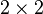$2 \times 2$ Rubik's cube. It can be opened and put together from different directions, thus creating a visually interesting effect.

## Construction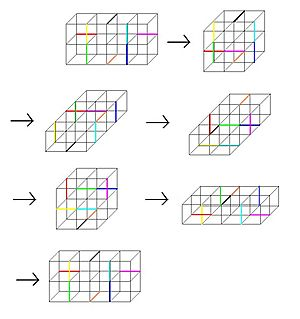The dark colored line segments are the taped position, while the light black line segments are not taped. From the 6 cycle state of the Infinity cube, you can see that its group representation is isomorphic to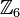$\mathbb Z_6$.

The principle of the Infinity Cube is simple and can be made by hand with simple paper cutting and pasting. First make 8 small squares, then arrange the small squares in a 2 by 2 by 2 way, and tape 8 edges together. When combined, there are 24 small squares exposed and 24 small squares hidden inside.

## Mathematics

Like the Rubik's Cube, the various states of the Infinity cube can be represented as a group, but the Infinity cube have far fewer variations than the Rubik's Cube, so the representation is also much simpler.

Rubik's Cube group have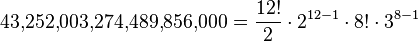$43{,}252{,}003{,}274{,}489{,}856{,}000\,\! = \frac{12!}{2} \cdot 2^{12-1} \cdot 8! \cdot 3^{8 - 1}$ elements，and isomorphic to the below group，where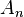$A_n$ are alternating groups and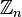$\mathbb Z_n$ are cyclic groups：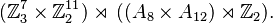$(\mathbb Z_3^7 \times \mathbb Z_2^{11}) \rtimes \,((A_8 \times A_{12}) \rtimes \mathbb Z_2).$

The largest group representation for Infinity cube only contains 6 elements, and can be represented as:$\mathbb Z_6$Infinity Cube Facts for Kids. Kiddle Encyclopedia.